# Data analysis using Pandas

• Difficulty Level : Medium
• Last Updated : 15 Oct, 2020

Pandas is the most popular python library that is used for data analysis. It provides highly optimized performance with back-end source code is purely written in C or Python.

```We can analyze data in pandas with:

SeriesDataFrames```

### Series:

Series is one dimensional(1-D) array defined in pandas that can be used to store any data type.

Code #1: Creating Series

 `# Program to create series`` ` `# Import Panda Library``import` `pandas as pd  `` ` `# Create series with Data, and Index``a ``=` `pd.Series(Data, index ``=` `Index)  `

Here, Data can be:

1. A Scalar value which can be integerValue, string
2. A Python Dictionary which can be Key, Value pair
3. A Ndarray

Note: Index by default is from 0, 1, 2, …(n-1) where n is length of data.

Code #2: When Data contains scalar values

 `# Program to Create series with scalar values `` ` `# Numeric data``Data ``=``[``1``, ``3``, ``4``, ``5``, ``6``, ``2``, ``9``]  `` ` `# Creating series with default index values``s ``=` `pd.Series(Data)    `` ` `# predefined index values``Index ``=``[``'a'``, ``'b'``, ``'c'``, ``'d'``, ``'e'``, ``'f'``, ``'g'``] `` ` `# Creating series with predefined index values``si ``=` `pd.Series(Data, Index) `

Output:

```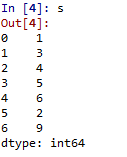Scalar Data with default Index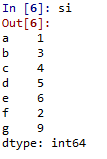Scalar Data with Index
```

Code #3: When Data contains Dictionary

 `# Program to Create Dictionary series``dictionary ``=``{``'a'``:``1``, ``'b'``:``2``, ``'c'``:``3``, ``'d'``:``4``, ``'e'``:``5``} `` ` `# Creating series of Dictionary type``sd ``=` `pd.Series(dictionary) `

Output:

```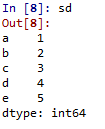Dictionary type data
```

Code #4:When Data contains Ndarray

 `# Program to Create ndarray series`` ` `# Defining 2darray``Data ``=``[[``2``, ``3``, ``4``], [``5``, ``6``, ``7``]]  `` ` `# Creating series of 2darray``snd ``=` `pd.Series(Data)    `

Output:

```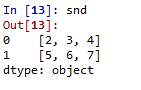Data as Ndarray
```

### DataFrames:

DataFrames is two-dimensional(2-D) data structure defined in pandas which consists of rows and columns.

Code #1: Creation of DataFrame

 `# Program to Create DataFrame`` ` `# Import Library``import` `pandas as pd   `` ` `# Create DataFrame with Data``a ``=` `pd.DataFrame(Data)  `

Here, Data can be:

1. One or more dictionaries
2. One or more Series
3. 2D-numpy Ndarray

Code #2: When Data is Dictionaries

 `# Program to Create Data Frame with two dictionaries`` ` `# Define Dictionary 1``dict1 ``=``{``'a'``:``1``, ``'b'``:``2``, ``'c'``:``3``, ``'d'``:``4``}   `` ` `# Define Dictionary 2     ``dict2 ``=``{``'a'``:``5``, ``'b'``:``6``, ``'c'``:``7``, ``'d'``:``8``, ``'e'``:``9``} `` ` `# Define Data with dict1 and dict2``Data ``=` `{``'first'``:dict1, ``'second'``:dict2} `` ` `# Create DataFrame ``df ``=` `pd.DataFrame(Data)  `

Output:

```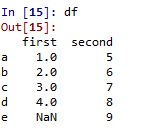DataFrame with two dictionaries
```

Code #3: When Data is Series

 `# Program to create Dataframe of three series ``import` `pandas as pd`` ` `# Define series 1``s1 ``=` `pd.Series([``1``, ``3``, ``4``, ``5``, ``6``, ``2``, ``9``])   `` ` `# Define series 2       ``s2 ``=` `pd.Series([``1.1``, ``3.5``, ``4.7``, ``5.8``, ``2.9``, ``9.3``]) `` ` `# Define series 3``s3 ``=` `pd.Series([``'a'``, ``'b'``, ``'c'``, ``'d'``, ``'e'``])     `` ` `# Define Data``Data ``=``{``'first'``:s1, ``'second'``:s2, ``'third'``:s3} `` ` `# Create DataFrame``dfseries ``=` `pd.DataFrame(Data)              `

Output:

```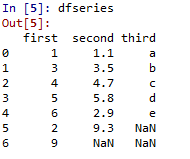DataFrame with three series
```

Code #4: When Data is 2D-numpy ndarray
Note: One constraint has to be maintained while creating DataFrame of 2D arrays – Dimensions of 2D array must be same.

 `# Program to create DataFrame from 2D array`` ` `# Import Library``import` `pandas as pd `` ` `# Define 2d array 1``d1 ``=``[[``2``, ``3``, ``4``], [``5``, ``6``, ``7``]] `` ` `# Define 2d array 2``d2 ``=``[[``2``, ``4``, ``8``], [``1``, ``3``, ``9``]] `` ` `# Define Data``Data ``=``{``'first'``: d1, ``'second'``: d2}  `` ` `# Create DataFrame``df2d ``=` `pd.DataFrame(Data)    `

Output:

```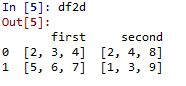DataFrame with 2d ndarray
```

My Personal Notes arrow_drop_up